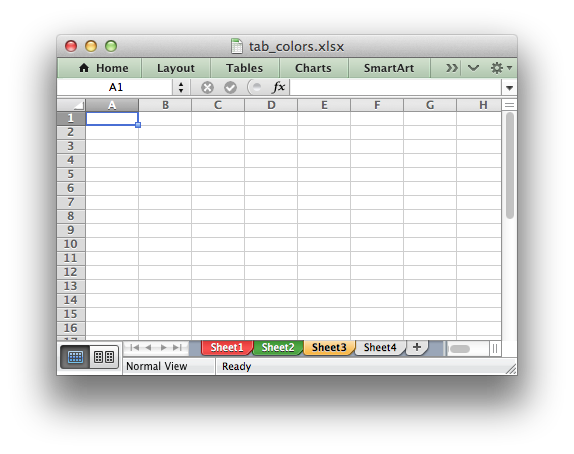libxlsxwriter
tab_colors.c

Example of how to set Excel worksheet tab colors./*
* Example of how to set Excel worksheet tab colors using libxlsxwriter.
*
* Copyright 2014-2021, John McNamara, jmcnamara@cpan.org
*
*/
#include "xlsxwriter.h"
int main() {
lxw_workbook *workbook = workbook_new("tab_colors.xlsx");
/* Set up some worksheets. */
lxw_worksheet *worksheet1 = workbook_add_worksheet(workbook, NULL);
lxw_worksheet *worksheet2 = workbook_add_worksheet(workbook, NULL);
lxw_worksheet *worksheet3 = workbook_add_worksheet(workbook, NULL);
lxw_worksheet *worksheet4 = workbook_add_worksheet(workbook, NULL);
/* Set the tab colors. */
worksheet_set_tab_color(worksheet3, 0xFF9900); /* Orange. */
/* worksheet4 will have the default color. */
worksheet_write_string(worksheet4, 0, 0, "Hello", NULL);
workbook_close(workbook);
return 0;
}
workbook_close
lxw_error workbook_close(lxw_workbook *workbook)
Close the Workbook object and write the XLSX file.
workbook_new
lxw_workbook * workbook_new(const char *filename)
Create a new workbook object.
lxw_worksheet
Struct to represent an Excel worksheet.
Definition: worksheet.h:2107
LXW_COLOR_GREEN
@ LXW_COLOR_GREEN
Definition: format.h:196
lxw_workbook
Struct to represent an Excel workbook.
Definition: workbook.h:280
worksheet_set_tab_color
void worksheet_set_tab_color(lxw_worksheet *worksheet, lxw_color_t color)
Set the color of the worksheet tab.
worksheet_write_string
lxw_error worksheet_write_string(lxw_worksheet *worksheet, lxw_row_t row, lxw_col_t col, const char *string, lxw_format *format)
Write a string to a worksheet cell.
LXW_COLOR_RED
@ LXW_COLOR_RED
Definition: format.h:217